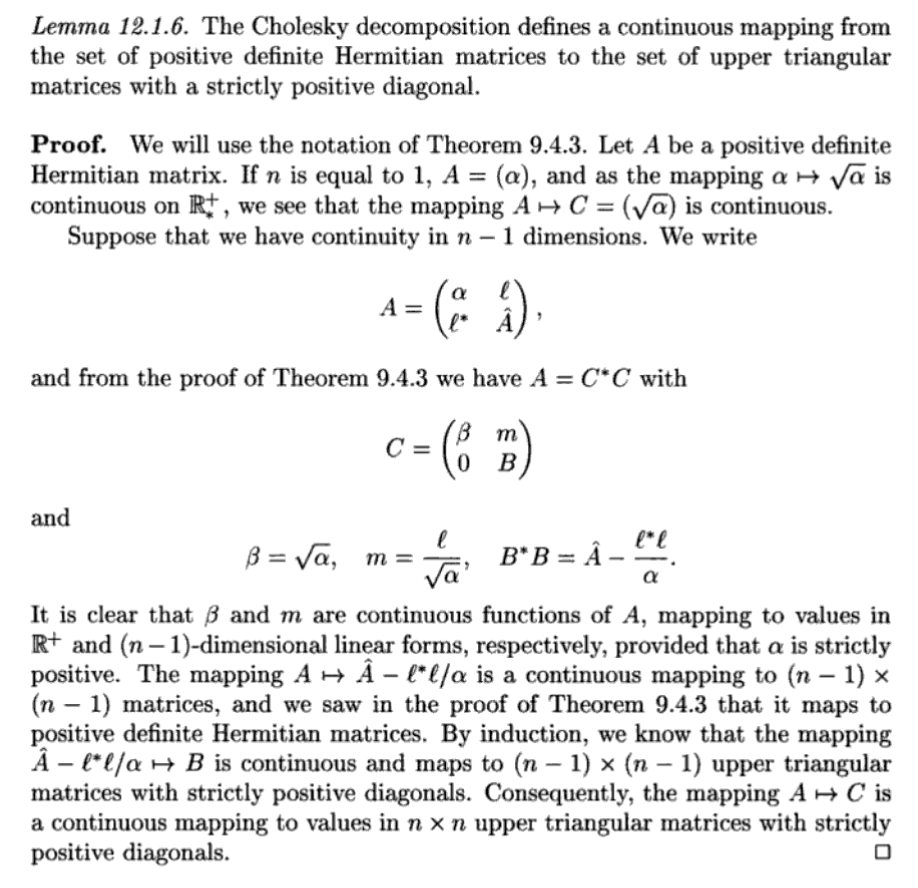# Reference request: continuity of Cholesky factor

It most books dealing with Cholesky decomposition, or it is variants, one finds a statement of the form if $$A$$ is symmetric $$k\times k$$ positive semi-definite (non-negative definite) then the $$k\times k$$ matrix $$L$$ solving $$A=RR^{\top}.$$ Note: I do not require that $$A$$ is positive definite, so $$A^{-1}$$ may not exist. However, I do require that it is symmetric.

Following his post, we see that under additional constraints there is a unique choice

Theorem 10.9. Let $$A\in\mathbb R^{n\times n}$$ be positive semidefinite of rank $$r$$. (a) There exists at least one upper triangular $$R\in\mathbb R^{n\times n}$$ with nonnegative diagonal elements such that $$A = R^TR$$. (b) There is a permutation $$\Pi$$ such that $$\Pi^TA\Pi$$ has a unique Cholesky factorization, which takes the form $$\Pi^TA\Pi=R^TR,\quad R=\left(\begin{matrix} R_{11} & R_{12} \\ 0 & 0\end{matrix}\right),$$ where $$R_{11}$$ is $$r \times r$$ upper triangular with positive diagonal elements.

However, I cannot find the source of book or paper saying map $$A \to R$$ is continuous.

• I have edited the title. The matrix square root is another thing, and proving that it is continuous is nontrivial (for non-symmetric matrices) in my view. May 25, 2020 at 9:55
• @FedericoPoloni What do you mean? In the case of semi-definite, ut symmetric matrices, would it be easier?
– ABIM
May 25, 2020 at 9:56
• There is a thing called "the (principal) matrix square root", which is defined for all matrices (possibly nonsymmetric) with no real negative eigenvalues and no nontrivial Jordan blocks in zero. It is continuous, but it is nontrivial to prove it. However, it is not what you are asking about here, so I have changed the title. Calling a Cholesky factor "square root" is slightly improper, although I have already heard it in various contexts. May 25, 2020 at 10:26
• No worries, I worked out what I needed from the answer as you pointed out. However, now I'm interested (purely out of curiousity) do you have a reference to this principle matrix squre-root s, purely our of scientific interest.
– ABIM
May 25, 2020 at 10:36
• Higham's book Functions of matrices. It has a definition on how to extend an arbitrary scalar function to matrices (like you probably already studied with the exponential of a nonsymmetric matrix) in Chapter 1, including some remarks on branches and the principal square root, and then a chapter devoted to the properties of matrix square roots. May 25, 2020 at 11:04

A subtle issue is that $$\Pi$$ is not unique here. For instance, if

$$A = \begin{bmatrix} 1 & 0 & 0\\\\ 0 & 0 & 0\\\\ 0 & 0 & 0 \end{bmatrix}$$

then you can take both the identity and $$(23)$$ as the permutation. Similarly, if $$A=I$$, then any $$\Pi$$ will work (and $$R=I$$).

I don't think you can speak about continuity until you resolve this ambiguity.

• How can it be resolved?
– ABIM
May 25, 2020 at 11:44
• It's up to you to define which decomposition exactly you need! May 25, 2020 at 11:57
• But are there reasonable known criteria on a decomposition, or choice of $\Pi$ as a function of $A$, making the map $A\to \mbox{ Cholesky factor of A}$ continuous? For example with the requirement that $\|A -R\|_F$ be minimal be such a requirement? (I've never had this type of issue before, so I hope my question is not too misguided).
– ABIM
May 25, 2020 at 12:15
• I am not sure if one can come up with a deterministic criterion for $\Pi$ that solves this problem. For instance, if $A_{2n} = diag(1,1/n,0)$, $A_{2n+1}=diag(1,0,1/n)$, then it seems that one needs to choose the identity permutation on even indices and (23) on odd indices. So the sequence $A_{n}$ converges to the matrix $A$ in my answer, but $\Pi$ is not eventually constant. (The factors $R_n$ converge to $R$, though, so maybe there is a way out.) I don't have a ready answer, sorry. May 25, 2020 at 12:36
• How do you plan to use this inverse? That is not clear to me. What is "this space"? May 26, 2020 at 8:38• That only takes care of the positive definite case. I think the real issue that the question raises is about allowing zero eigenvalues. May 25, 2020 at 13:06